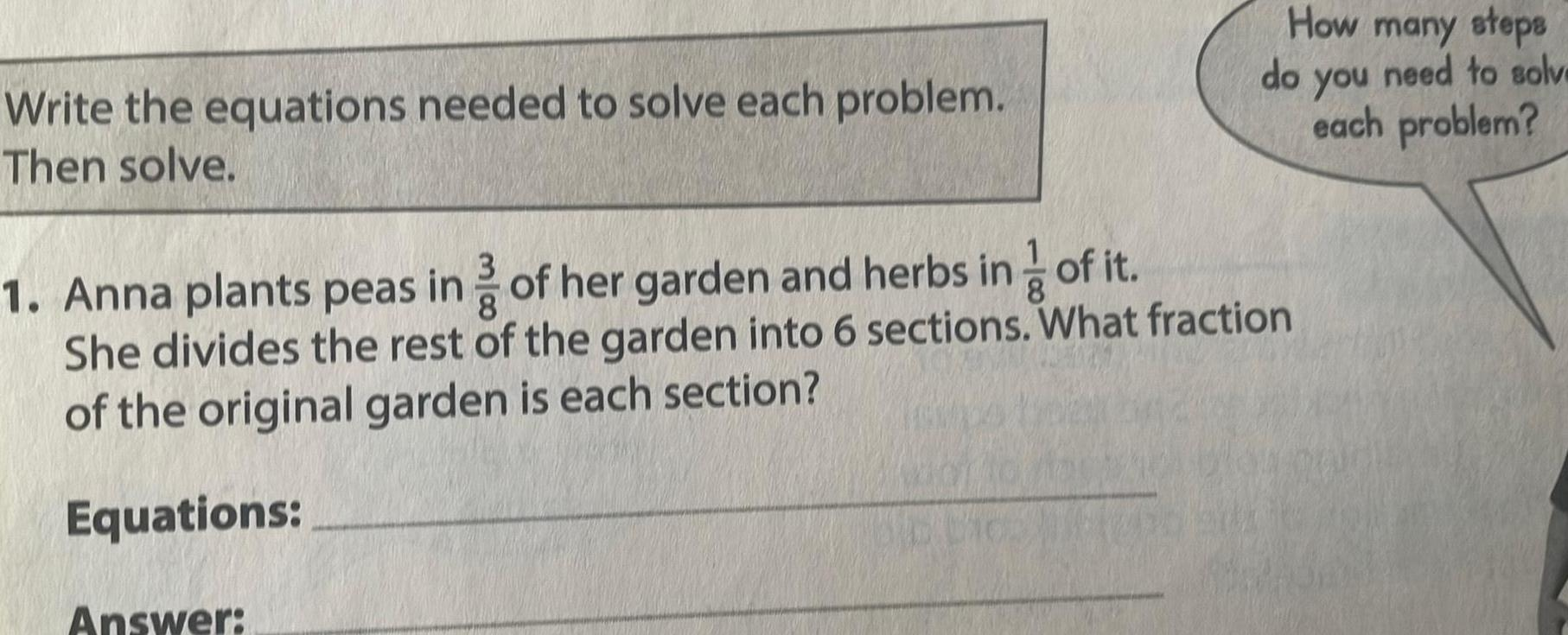Question:

# Write the equations needed to solve each problem Then solve

Last updated: 5/11/2023Write the equations needed to solve each problem Then solve How many steps Answer do 1 Anna plants peas in of her garden and herbs in of it She divides the rest of the garden into 6 sections What fraction of the original garden is each section Equations need to solve you each problem